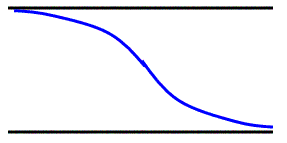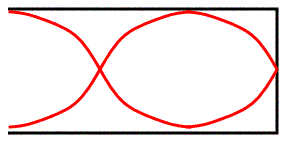# An open pipe has a length of 0.75 m. What would be the length of a closed organ pipe whose third harmonic(n=3) is the same as the fundamental frequency of the open pipe?

Sep 14, 2015

#### Answer:

$3.4 \text{m}$

#### Explanation:

For an open pipe the 1st harmonic looks like this:This refers to half a wavelength so:

${L}_{1} = \frac{\lambda}{2}$

$\lambda = 2 {L}_{1}$

Since $v = f \lambda$:

$f = \frac{v}{\lambda} = \frac{v}{2 {L}_{1}}$$\text{ }$$\textcolor{red}{\left(1\right)}$

For the closed pipe the 3rd harmonic looks like this:In this case:

${L}_{2} = \frac{3}{4} \lambda$

$\lambda = \frac{4 {L}_{2}}{3}$

So $v = f . \frac{4 {L}_{2}}{3}$

So $f = \frac{3 v}{4 {L}_{2}}$ $\text{ }$$\textcolor{red}{\left(2\right)}$

These frequencies are equal so putting $\textcolor{red}{\left(1\right)}$ equal to $\textcolor{red}{\left(2\right)} \Rightarrow$

$\frac{v}{2 {L}_{1}} = \frac{3 v}{4 {L}_{2}}$

$\frac{3}{4 {L}_{2}} = \frac{1}{2 {L}_{1}}$

${L}_{2} = \frac{6 {L}_{1}}{4}$

${L}_{2} = \frac{3 \times 6 \times 0.75}{4}$

${L}_{2} = 3.4 \text{m}$